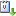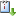Usted está aquí: Existence and nonexistence results for a class of asymptotically linear non-autonomous equations in $$\mathbb{R}^N$$

# Existence and nonexistence results for a class of asymptotically linear non-autonomous equations in $$\mathbb{R}^N$$

Liliane A. Maia (Universidad de Brasilia)- Jueves 4 de abril de 2013, 11:00 hrs.
Ponente:
Cuándo 04/04/2013 de 12:00 a 13:00 Graciela SalicrupvCaliCal

We will present some recent results on the existence of solutions for a class of elliptic equations which are asymptotically linear at infinity$-\Delta u + V(x) u = a(x) f(u) \quad\in \mathbb{R}^n,$  where $$0 < V(x)\to V_\infty$$   and $$0 < a(x)\to a_\infty$$,  as  $$|x|\to\infty, V_\infty < a_\infty$$  and $$f(s)/s \to 1$$ as $$s \to \infty$$. Using  concentration compactness arguments and a general Pohozaev type manifold, we find bound state solutions via a linking theorem.

Moreover, we show that a minimizing problem, related to the existence of a ground state, has no solution.
This is a work in collaboration with Raquel Lehrer from Universidade Estadual do Oeste do Paraná- UNIOESTE, Campus Cascavel.